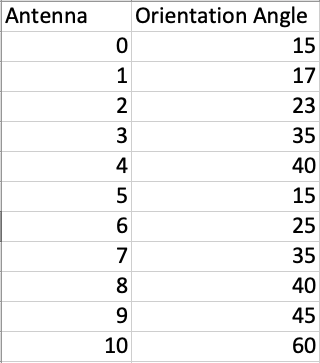# Change The Pandas.series Elements Using Apply And Transform

## Overview:

• The contents of a pandas.Series instance can be transformed based on a mathematical function.
• By calling Series.apply() method and passing either a Python function or a numpy universal function(ufunc) as the value for func parameter, the values present in the series are transformed by the applied/passed-in function.
• The Series.trasnform() method is more versatile through accepting a function name, a list of function names, or a function that works with Series.Apply().

## Example - Using Series.apply():

• The example Python code reads a CSV file (antennas.csv) and loads the columns into a DataFrame.• The column containing the orientation angles is selected into a Series and apply() is called by passing the math.cos() function as the parameter. This returns a new pandas.Series with cosine values of the orientation angles.
 # Example Python program to transform the values # of a pandas.series instance by applying a function import pandas as pds import math   # Load the data from the cake.csv dataFile = "./Antennas.csv"; df       = pds.read_csv(dataFile); series   = df["Orientation Angle"]; cosineValues = series.apply(math.cos); print(cosineValues);

## Output:

 0    -0.759688 1    -0.275163 2    -0.532833 3    -0.903692 4    -0.666938 5    -0.759688 6     0.991203 7    -0.903692 8    -0.666938 9     0.525322 10   -0.952413 Name: Orientation Angle, dtype: float64

## Example - Using Series.transform():

When more than one function is passed to transform() the result is a pandas.DataFrame whose columns are produced by the functions passed in.